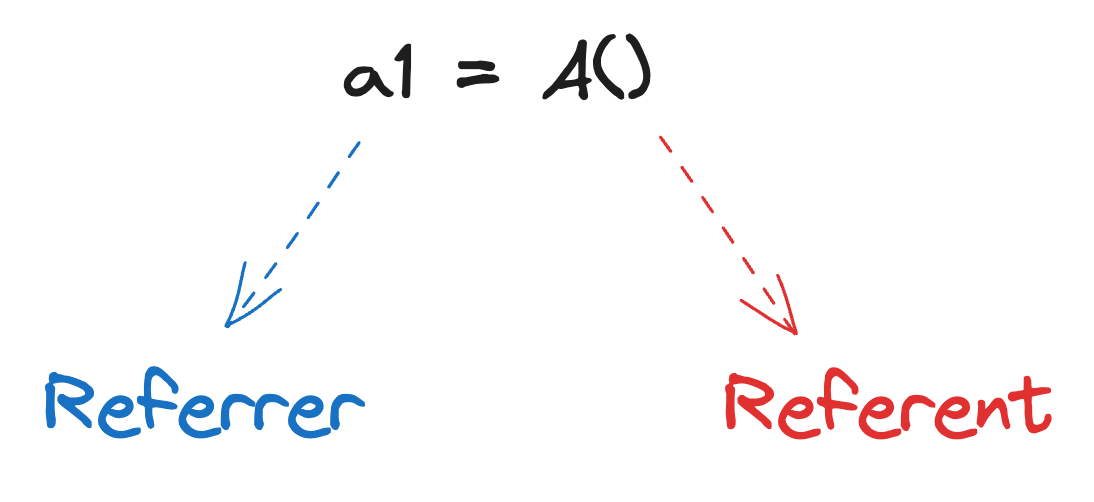# Python - 用範例學 weakref 模組

Posted on  Sep 6, 2023  by  Amo Chen  ‐ 7 min read

Python 是 1 個有垃圾回收機制(garbage collection, 或簡稱 GC)的程式語言，簡而言之是一種自動的記憶體管理機制，當某些記憶體空間沒有任何程式用到時，就會被回收，然後釋放這些記憶體空間，避免記憶體越用越少，最後導致程式錯誤、無法執行等問題。

GC 機制是為了減少開發者的負擔，例如 C 語言就需要手動釋放記憶體空間（詳細請參閱 free() 函式），所以忘記釋放記憶體空間造成記憶體洩漏(memory leak)的問題屢見不鮮，但如果交給 GC 的話，就可以讓開發者不太需要考慮記憶體管理的問題，增加開發的效率也降低犯錯的機率。

• Python 3

### Reference Counting #

Python Reference counting 的大致運作方式，是每個物件實例(instance)都會有一個計數器，當有對象參照到某一個物件實例時，該物件實例的計數器就會加 1 ，如果不再參照到就會減 1 ，當這個計數器數值為零時，就會被 GC 機制回收，並釋放記憶體空間。

``````import gc

class A: pass

a1 = A()
``````### 取得 Reference Count #

``````import sys

class A: pass

a1 = A()

print('After `a1 = A()` =>', sys.getrefcount(a1))

a2 = a1

print('After `a2 = a1` =>', sys.getrefcount(a1))

a2 = None

print('After `a2 = None` =>', sys.getrefcount(a1))
``````

``````After `a1 = A()` => 2
After `a2 = a1` => 3
After `a2 = None` => 2
``````

Return the reference count of the object. The count returned is generally one higher than you might expect, because it includes the (temporary) reference as an argument to getrefcount().

``````>>> class A:
>>> ...     def __del__(self):
>>> ...             print('Garbage collected!', self)
>>> ...
>>> A()
>>> <__main__.A object at 0x10153c550>
>>> _
>>> <__main__.A object at 0x10153c550>
>>> 3
>>> Garbage collected! <__main__.A object at 0x10153c550>
>>>
``````

``````class A:
def __del__(self):
print('Garbage collected!', self)

A()

print('---end---')
``````

``````Garbage collected! <__main__.A object at 0x1049cbfa0>
---end---
``````

### 強參照(Strong Reference) #

In Python’s C API, a strong reference is a reference to an object which is owned by the code holding the reference. The strong reference is taken by calling Py_INCREF() when the reference is created and released with Py_DECREF() when the reference is deleted.

Python 預設使用的參照方式是強參照(strong reference)，這種參照方式如前述般會對物件實例的計數器有 +1 的效果，解除參照時則會有 -1 的效果。

``````from pprint import pprint

class Image:
def __init__(self, key):
self.key = key
self.body = f'body of {key}'

def __del__(self):
print('Garbage collected!', self.key, self)

CACHE = {}

def main():
a = Image('a')
CACHE[a.key] = a

b = Image('b')
CACHE[b.key] = b

c = Image('c')
CACHE[c.key] = c

b = None
print('CACHE:')
pprint(CACHE)
print('--- end of main() ---')

if __name__ == '__main__':
main()
print('--- end ---')
``````

``````CACHE:
{'a': <__main__.Image object at 0x103443fa0>,
'b': <__main__.Image object at 0x103443d00>,
'c': <__main__.Image object at 0x1034ac850>}
--- end of main() ---
--- end ---
Garbage collected! b <__main__.Image object at 0x103443d00>
Garbage collected! c <__main__.Image object at 0x1034ac850>
Garbage collected! a <__main__.Image object at 0x103443fa0>
``````

``````class Image:
def __init__(self, key):
self.key = key
self.body = f'body of {key}'

def __del__(self):
print('Garbage collected!', self.key, self)

CACHE = {}

def main():
a = Image('a')
CACHE[a.key] = a

b = Image('b')
CACHE[b.key] = b

c = Image('c')
CACHE[c.key] = c

del CACHE[b.key]
b = None
print('--- end of main() ---')

if __name__ == '__main__':
main()
print('--- end ---')
``````

``````Garbage collected! b <__main__.Image object at 0x104d88850>
--- end of main() ---
--- end ---
Garbage collected! a <__main__.Image object at 0x104d54550>
Garbage collected! c <__main__.Image object at 0x104d88a00>
``````

### 弱參照(weak reference) #

``````>>> import sys, weakref
>>> class A: pass
...
>>> a = A()
>>> sys.getrefcount(a)
2
>>> b = weakref.ref(a)
>>> sys.getrefcount(a)
2
>>> c = weakref.ref(a)
>>> sys.getrefcount(a)
2
``````

``````import weakref

from pprint import pprint

class Image:
def __init__(self, key):
self.key = key
self.body = f'body of {key}'

def __del__(self):
print('Garbage collected!', self.key, self)

CACHE = {}

def main():
a = Image('a')
CACHE[a.key] = weakref.ref(a)

b = Image('b')
CACHE[b.key] = weakref.ref(b)

c = Image('c')
CACHE[c.key] = weakref.ref(c)

b = None
print('CACHE:')
pprint(CACHE)
print('--- end of main() ---')

if __name__ == '__main__':
main()
print('--- end ---')
``````

``````Garbage collected! b <__main__.Image object at 0x102bc3d00>
CACHE:
{'a': <weakref at 0x102c4b810; to 'Image' at 0x102bc3fa0>,
'c': <weakref at 0x102c2cdb0; to 'Image' at 0x102c239d0>}
--- end of main() ---
Garbage collected! a <__main__.Image object at 0x102bc3fa0>
Garbage collected! c <__main__.Image object at 0x102c239d0>
--- end ---
``````

``````cache_ref = CACHE['b']
value = cache_ref()
if value is None:
print('No cache')
else:
print('Got cache', value)
``````

### WeakValueDictionary #

``````import weakref

from pprint import pprint

class Image:
def __init__(self, key):
self.key = key
self.body = f'body of {key}'

def __del__(self):
print('Garbage collected!', self.key, self)

CACHE = weakref.WeakValueDictionary()

def main():
a = Image('a')
CACHE[a.key] = a

b = Image('b')
CACHE[b.key] = b

c = Image('c')
CACHE[c.key] = c

b = None
print('CACHE:')
pprint(list(CACHE.items()))
print('--- end of main() ---')

if __name__ == '__main__':
main()
print('--- end ---')
``````

``````Garbage collected! b <__main__.Image object at 0x100f5c940>
CACHE:
[('a', <__main__.Image object at 0x100ef3d00>),
('c', <__main__.Image object at 0x100f9f3a0>)]
--- end of main() ---
Garbage collected! a <__main__.Image object at 0x100ef3d00>
Garbage collected! c <__main__.Image object at 0x100f9f3a0>
--- end ---
``````

WeakValueDictionary 類似的還有 WeakKeyDictionary, WeakKeyDictionary 是 key 沒有任何強參照時，會自動清除 key 與 value 。

### Circular Reference #

``````import sys

class A:
def __init__(self, key):
self.a = self
self.key = key

def __del__(self):
print('Garbage collected!', self.key)

a = A('a')
print("A's refcount:", sys.getrefcount(a))
a = None

print('---end---')
``````

``````A's refcount: 3
---end---
Garbage collected! a
````````````import sys
import weakref

class A:
def __init__(self, key):
self.a = weakref.proxy(self)
self.key = key

def __del__(self):
print('Garbage collected!', self.key)

a = A('a')
print("A's refcount:", sys.getrefcount(a))
a = None

print('---end---')
``````

``````A's refcount: 2
Garbage collected! a
---end---
``````

### 總結 #

weakref 確實是 1 個不太好理解的功能，我自己也是爬梳很多文章、影片，透過實作範例加上寫成文章的過程終於才理解 weakref, 而這個功能也確實對於 Python 的 GC 機制相當重要，運用得當可以有效避免記憶體洩漏的問題。

Happy Coding!

sys — System-specific parameters and functions

weakref — Weak references

python: what is weakref? (intermediate - advanced) anthony explains #366